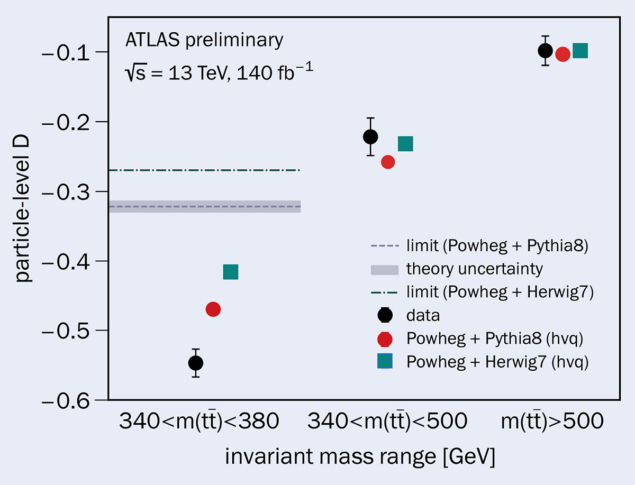# Highest-energy observation of quantum entanglement

29 September 2023

A report from the ATLAS experiment.Fig. 1. The observed and expected values of the entanglement witness D for different regions defined by mtt, compared to various Monte Carlo predictions. The value of the entanglement limit D = –1/3 at parton level is converted to particle level. Source: ATLAS-CONF-2023-069

Entanglement is an extraordinary feature of quantum mechanics: if two particles are entangled, the state of one particle cannot be described independently from the other. It has been observed in a wide variety of systems, ranging from microscopic particles such as photons or atoms to macroscopic diamonds, and over distances ranging from the nanoscale to hundreds of kilometres. Until now, however, entanglement has remained largely unexplored at the high energies accessible at hadron colliders, such as the LHC.

At the TOP 2023 workshop, which took place in Michigan this week, the ATLAS collaboration reported a measurement of entanglement using top-quark pairs with one electron and one muon in the final state selected from proton–proton collision data collected during LHC Run 2 at a centre-of-mass energy of 13 TeV, opening new ways to test the fundamental properties of quantum mechanics.

Two-qubit system
The simplest system which gives rise to entanglement is a pair of qubits, as in the case of two spin-1/2 particles. Since top quarks are typically generated in top-antitop pairs (tt) at the LHC, they represent a unique high-energy example of such a two-qubit system. The extremely short lifetime of the top (10-25 s, which is shorter than the timescale for hadronisation and spin decorrelation) means that its spin information is directly transferred to its decay products. Close to threshold, the tt pair produced through gluon fusion is almost in a spin-singlet state, maximally entangled. By measuring the angular distributions of the tt decay products close to threshold, one can therefore conclude whether the tt pair is in an entangled state.

For this purpose, a single observable can be used as an entanglement witness, D. This can be measured from the distribution of cos𝜑, where 𝜑 is the angle between the charged lepton directions in each of the parent top and anti-top rest frames, with D = −3⋅⟨cos𝜑⟩. The entanglement criterion is given by D = tr(C)/3 < −1/3, where tr(C) is the sum of the diagonal elements of the spin-correlation matrix C of the tt̄ pair before hadronisation effects occur. Intuitively, this criterion can be understood from the fact that tr(C) is the expectation value of the product of the spin polarizations, tr(C) =〈σ⋅σ〉, with σ, σ being the t,t polarizations, respectively (classically tr(C) ≤ 1, since spin polarizations are unit vectors).  D is measured in a region where the invariant mass is approximately twice the mass of the top quark, 340 < mtt < 380 GeV, and is performed at particle level, after hadronisation effects occur.

This constitutes the first observation of entanglement between a pair of quarks and the highest-energy measurement of entanglement

The shape of cos𝜑 is distorted by detector and event-selection effects for which it has to be corrected. A calibration curve connecting the value of D before and after the event reconstruction is extracted from simulation and used to derive D from the corresponding measurement, which is then compared to predictions from state-of-the-art Monte Carlo simulations. The measured value D = -0.547 ± 0.002 (stat.) ± 0.021 (syst.) is well beyond 5σ from the non-entanglement hypothesis. This constitutes the first-ever observation of entanglement between a pair of quarks and the highest-energy measurement of entanglement.

Apart from the intrinsic interest of testing entanglement under unprecedented conditions, this measurement paves the way to use the LHC as a novel facility to study quantum information. Prime examples are quantum discord, which is the most basic form of quantum correlations; quantum steering, which is how one subsystem can steer the state of the other one; and tests of Bell’s inequalities, which explore non-locality.  Furthermore, borrowing concepts from quantum information theory inspires new approaches to search for physics beyond the Standard Model.